# UMDCTF 2023 - Pokeptcha

##### by not_really

Team Rocket keeps taking down my website! I’m testing out this new type of captcha, but it doesn’t seem to be working as expected. None of the choices are valid! Can you solve it for me?

By: umasiPoKePTCHA is an obfuscated JavaScript challenge with a picture of the “jigglypuff seen from above” scene, three buttons, and an “other” textbox to submit with.

Let’s take a look at the JavaScript on the page.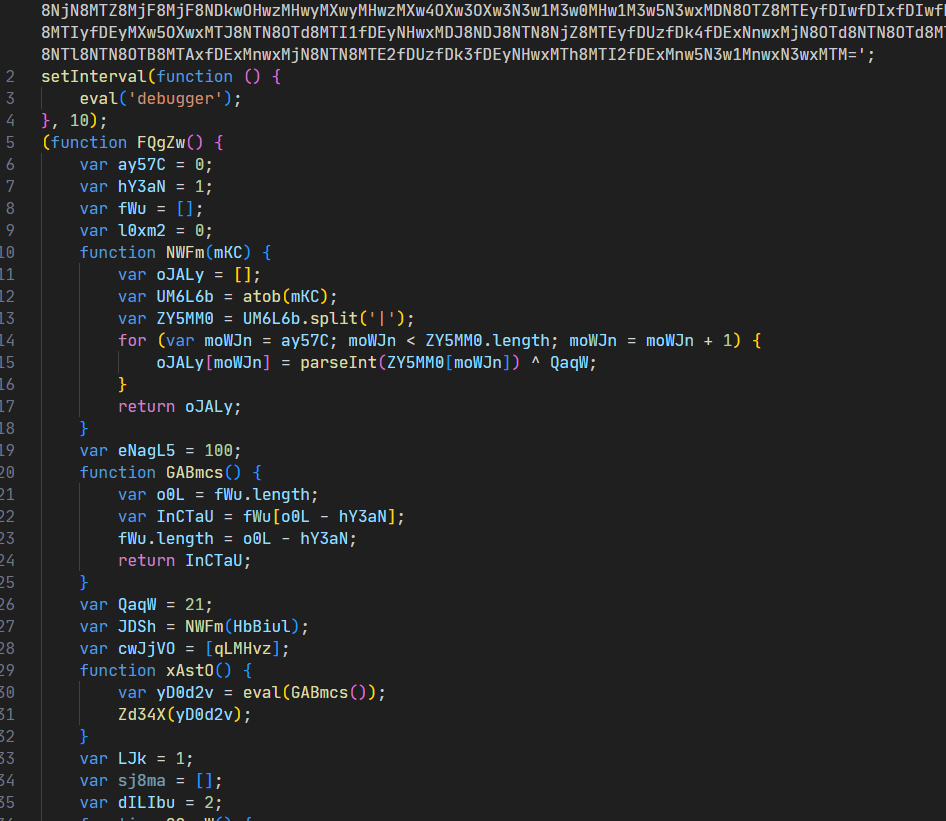Doesn’t seem to be any obfuscator I’ve seen before, so it’s probably just some renaming. Let’s take a look at where the input comes in at. Normally I would say to start at the input and work through it, but in this case it would be better to clean up some of the code. For example, `var eNagL5 = 100;` in the above code is only used for comparisons and never assigned later, so it should be inlined into the functions they’re used in. For variables that are assigned later on, we can put them next to the right function instead of the random locations they seem to be right now (like how `QaqW` at line 26 is only used on line 15.) After cleaning it up a bit and putting similar functions together, we get code more like this:

``````    var fWu = []; // stack?
var LZX = false; // success?
var sj8ma = []; // unused?
var l0xm2 = 0; // program data counter

function KmyVf() { // reset
fWu = [];
LZX = false;
sj8ma = [];
l0xm2 = 0;
}

function NWFm(mKC) { // decrypt HbBiul
var oJALy = [];
var UM6L6b = atob(mKC);
var ZY5MM0 = UM6L6b.split('|');
for (var i = 0; i < ZY5MM0.length; i++) {
oJALy[i] = parseInt(ZY5MM0[i]) ^ 21;
}
return oJALy;
}

var JDSh = NWFm(HbBiul); // program data

function OGmoW() { // get next index for program data
l0xm2 = l0xm2 + 1;
return l0xm2 - 1;
}

function jHv8H() { // read string from program data
var HUD = OGmoW();
var j4r = JDSh[HUD];
var XmCf = '';
for (var e4uNJ = 0; e4uNJ < j4r; e4uNJ = e4uNJ + 1) {
HUD = OGmoW();
XmCf = XmCf + String.fromCharCode(JDSh[HUD]);
}
return XmCf;
}

function GABmcs() { // pop item from fWu
var o0L = fWu.length;
var InCTaU = fWu[o0L - 1];
fWu.length = o0L - 1;
return InCTaU;
}

function Zd34X(LtmHw) { // push item to fWu
var ZkwpV6 = fWu.length;
fWu[ZkwpV6] = LtmHw;
}

function OpHnMw() { // read number from program data
var nOeyBy = OGmoW();
return JDSh[nOeyBy];
}

var QZOR;
var cwJjVO = [qLMHvz, xAstO, A7G];
function LRRZ(input) { // run
KmyVf();
fWu.push(input);
QZOR = Date.now();
while (1 < 100) {
var Mwcow = OpHnMw();
if (Mwcow == 100) {
sj8ma = [];
return LZX;
}
var lAG1Gj = cwJjVO[Mwcow];
if (lAG1Gj == 2) {
continue;
}
lAG1Gj();
}
KmyVf();
}

function qLMHvz() { // cwJjVO - read string or number and push into fWu
fWu.push(I13rI());
}

function I13rI() { // read string or number
var xLU = OGmoW();
var zujC = JDSh[xLU];
if (zujC & 1) {
var pjE2 = jHv8H();
return pjE2;
}
xLU = OGmoW();
return JDSh[xLU];
}

function xAstO() { // cwJjVO - pop fWu value and eval
var yD0d2v = eval(GABmcs());
Zd34X(yD0d2v);
}

function A7G() { // cwJjVO - pop three fWu values and assign
var nbP = [];
for (var ICa = 0; ICa < 3; ICa = ICa + 1) {
var ZyJG = GABmcs();
nbP.push(ZyJG);
}
pBpU0(...nbP);
}

function pBpU0(OiVSu, gstj, LPU6hP) { // assign item property
var C4VGI = { b: OiVSu };
LPU6hP[gstj] = C4VGI.b;
}

globalThis.run = LRRZ;
``````

After cleaning it up a little bit, it’s a bit more clear what’s happening. The big base64 string `HbBiul` is XOR “decrypted” into `JDSh` and it has three different instructions (which are defined in `cwJjVO`):

• Read an int or string from the program and push it onto `fWu` (the VM’s stack).
• Pop from the stack and eval it.
• Pop three values from the stack and assign `Z[Y] = X` where `X` is the first item popped and `Z` is the last.

The int/string encoding is simple. If the value read is a 0, it reads another number and returns it. If the value read is a 1, it reads a length value, then reads that many values and returns them as a string.

Seems simple, so I wrote a disassembler to see what it would look like.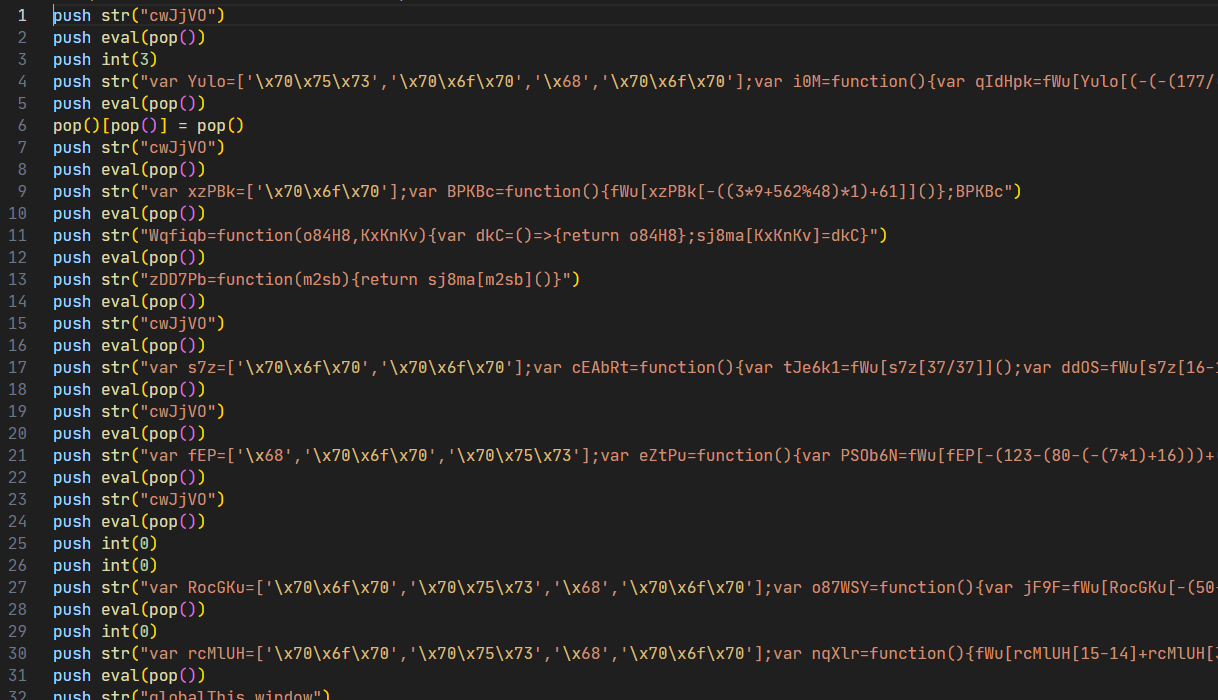Uh oh, evaling a lot of complicated JavaScript. So what’s it doing? Here’s the first string beautified:

``````var Yulo = ['pus', 'pop', 'h', 'pop'];
var i0M = function() {
var qIdHpk = fWu[Yulo[(-(-(177 / (59 * (91 / 91))) + (3000 / 50 - 1043 % 62)) + 7) * 1]]();
var j9M = fWu[Yulo[91 - (26 + (42 + 20))]]();
j9M[Yulo[0 / (87 - 118 % 34)] + Yulo[82 % 5]](qIdHpk)
};
i0M
``````

And after manually evaluating the math operations and inlining the strings:

``````var i0M = function() {
var qIdHpk = fWu['pop']();
var j9M = fWu['pop']();
j9M['push'](qIdHpk)
};
i0M
``````

So it’s popping two values from the stack and pushing to some array. What would be on the stack at this point?

``````push str("cwJjVO")
push eval(pop())
push int(3)
``````

`cwJjVO` (the list of functions used by the VM) and `3`. So it’s adding more instructions at runtime. Uh oh. This goes on for a while, so I had the idea to download the site, disable the simple debugger check, and check when `cwJjVO` changes size.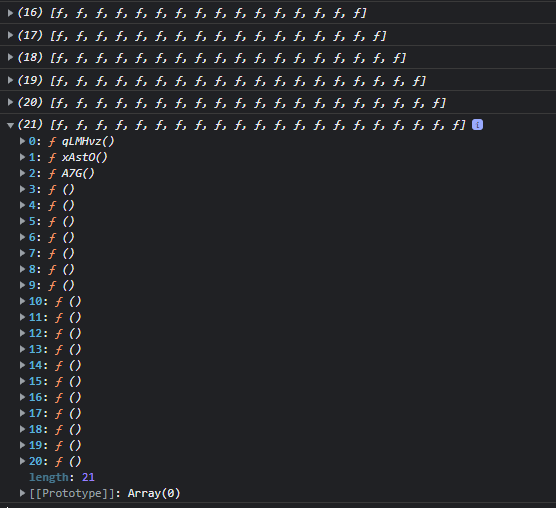I temporarily switched to Chrome at this point because it has a nice feature that let’s you view the code of any function by right clicking it in the console output (which somehow Firefox doesn’t have). It even formats it automatically!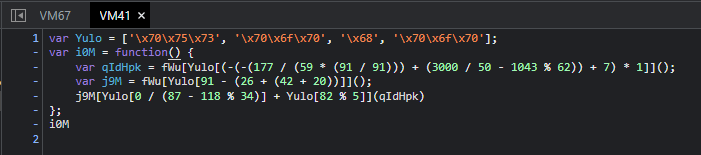I went through each one of these individually, copying them to a new text file and fixing the strings.

``````3 => function(){ // push to array
var qIdHpk=fWu["pop"]();
var j9M=fWu["pop"]();
j9M["push"](qIdHpk)
}
4 => function(){ // pop and discard value
fWu["pop"]()
}
Wqfiqb=function(o84H8,KxKnKv){
sj8ma[KxKnKv]=()=>{return o84H8};
}
5 => function(){ // put value into vm memory
var tJe6k1=fWu["pop"]();
var ddOS=fWu["pop"]();
Wqfiqb(ddOS,tJe6k1)
}
zDD7Pb=function(m2sb){
return sj8ma[m2sb]()
}
6 => function(){ // read memory at position
var PSOb6N=fWu["pop"]();
fWu["push"](zDD7Pb(PSOb6N))
}
7 => function(){ // subtract two values
var jF9F=fWu["pop"]();
var sA9E=fWu["pop"]();
fWu["push"](jF9F-sA9E)
}
8 => function(){ // or
fWu["push"](fWu["pop"]() || fWu["pop"]())
}
9 => function(){ // push global
fWu["push"](global)
}
10 => function(){ // push null
fWu["push"](null)
}
l0xm2=fWu["pop"]()
}
12 => function(){ // x.apply(y, z)
var OkEsZR=fWu["pop"]();
var ElaO=fWu["pop"]();
var bnw2qp=fWu["pop"]();
fWu["push"](OkEsZR["apply"](ElaO,bnw2qp))
}
13 => function(){ // push empty array
fWu["push"]([])
}
14 => function(){ // check if not equal
var lKj6yX=fWu["pop"]();
var gkI3Jh=fWu["pop"]();
var DdxPd2=fWu["pop"]();
l0xm2 = lKj6yX == gkI3Jh ? l0xm2 : DdxPd2
}
15 => function(){ // xor
var na6On=fWu["pop"]();
var bb6=fWu["pop"]();
fWu["push"](na6On^bb6)
}
16 => function(){ // modulo
var QtiLR=fWu["pop"]();
var NZ5hG=fWu["pop"]();
fWu["push"](QtiLR%NZ5hG)
}
17 => function(){ // X[Y]
var CeQp=fWu["pop"]();
var Bc2P=fWu["pop"]();
fWu["push"](CeQp[Bc2P])
}
var agy=fWu["pop"]();
var X1S=fWu["pop"]();
fWu["push"](agy+X1S)
}
19 => function(){ // shift right (tos1 >> tos0)
var qj27=fWu["pop"]();
var uzSl=fWu["pop"]();
fWu["push"](uzSl>>qj27)
}
20 => function(){ // check if equal
var nPqZy=fWu["pop"]();
var pAtzQ=fWu["pop"]();
var kWyvR=fWu["pop"]();
l0xm2 = nPqZy != pAtzQ ? kWyvR : l0xm2
}
``````

I started to write a stack based “decompiler” but I ended up running out of stack and figured there was something wrong with my code. So I did the rest of the challenge with the disassembly by manually replacing `push()` and `pop()`s. I fixed this after the competition ended by just adding another random item to the stack. I assumed my code was just wrong (and maybe it still is), but this seemed to do the trick. Here’s the (almost) full decompiled code:

``````  // new instruction setup above this
mem = eval("globalThis") || eval("globalThis.window")
//
mem = []
mem.push(eval("var LtTZ7p=function(){debugger};LtTZ7p();LtTZ7p"))
mem.push(5)
push(eval("setInterval").apply(null, mem))
pop()
mem = mem["location"]["hostname"]
mem = eval("FQgZw")
push(mem["toString"].apply(mem, []))
mem = mem + mem["toString"].apply(mem, [])
mem = mem["Date"]
push(mem["now"].apply(mem, []))
mem = mem + mem["now"].apply(mem, []) - eval("QZOR") >> 3
mem = []
mem.push(mem)
push(eval("md5").apply(null, mem))
mem = eval("md5").apply(null, mem)
pop()
mem = <<<THE INPUT>>>
mem = []
mem = 0
mem = 0
mem = ""
mem = 0
lbl_4388:
mem[mem] = mem
mem = 1 + mem
if 256 != mem jump 4388
mem = 0
lbl_4431:
mem = mem + mem[mem]
mem = mem % mem["length"]
mem = []
mem.push(mem)
push(mem["charCodeAt"].apply(mem, mem))
mem = mem + mem["charCodeAt"].apply(mem, mem)
mem = mem % 256
mem = mem[mem]
mem[mem] = mem[mem]
mem[mem] = mem
mem = 1 + mem
if 256 != mem jump 4431
mem = 0
mem = 0
mem = 0
lbl_4622:
mem = 1 + mem % 256
mem = mem + mem[mem] % 256
mem = mem[mem]
mem[mem] = mem[mem]
mem[mem] = mem
mem = []
mem.push(mem)
push(mem["charCodeAt"].apply(mem, mem))
mem = mem[mem[mem] + mem[mem] % 256] ^ mem["charCodeAt"].apply(mem, mem)
mem = []
mem.push(mem)
push(eval("String")["fromCharCode"].apply(null, mem))
mem = eval("String")["fromCharCode"].apply(null, mem)
mem = mem + mem
mem = 1 + mem
if mem["length"] != mem jump 4622
mem = []
mem.push(mem)
push(eval("window")["btoa"].apply(null, mem))
mem = eval("window")["btoa"].apply(null, mem)
jump(4976)
lbl_4921:
mem = []
mem.push(mem)
push(mem["charCodeAt"].apply(mem, mem))
if mem == mem["charCodeAt"].apply(mem, mem) jump mem
jump(mem)

lbl_4976:
mem = 6456
mem = 5008
mem = 0
mem = 103
jump(4921)
lbl_5008:
mem = 5033
mem = 1
mem = 53
jump(4921)
lbl_5033:
mem = 5058
mem = 2
mem = 43
// ... this goes on for a while
``````

I manually added the labels based on the non-stack based disassembler. Although it’s decently readable, we can do even better. Here it is in a typical JavaScript flow:

``````setInterval(eval("var LtTZ7p=function(){debugger};LtTZ7p();LtTZ7p"), 5)

varB = globalThis.location.hostname
varB += FQgZw.toString()
varE = globalThis.Date
varB += varE.now() - (QZOR >> 3)
varB = md5(varB)

varG = "<<<THE INPUT>>>"

varA = []
varH = 0
varI = 0
varJ = ""

varK = 0
for (; varK != 256; varK++) {
varA[varK] = varK
}

varK = 0
for (; varK != 256; varK++) {
varH += varA[varK]
varL = varK % varB.length
varH += varB.charCodeAt(varL)
varH %= 256
varI = varA[varK]
varA[varK] = varA[varH]
varA[varH] = varI
}

varK = 0
varH = 0
varM = 0
for (; varM != varG.length; varM++) {
varK = (varK + 1) % 256
varH += varA[varK] % 256
varI = varA[varK]
varA[varK] = varA[varH]
varA[varH] = varI
varO = varA[varA[varH] + varA[varK] % 256] ^ varG.charCodeAt(varM)
varP = String.fromCharCode(varO)
varJ += varP
}

varQ = window.btoa(varJ)
jump(4976)
lbl_4921:
if (varT == varQ.charCodeAt(varS)) jump(varU)
jump(varV)

lbl_4976:
varU = 6456
varV = 5008
varS = 0
varT = 103
jump(4921)
lbl_5008:
varV = 5033
varS = 1
varT = 53
jump(4921)
lbl_5033:
varV = 5058
varS = 2
varT = 43
// ...
``````

The final code isn’t too complicated. `varB` adds together the hostname (to check if you downloaded the webpage and are running it locally), the current date subtracted from `QZOR`’s date (to detect debugging), and the entire `FQgZw.toString()` contents (to check if you modified any of the code). This string is md5’d and used to scramble the sbox, `varA`. Then the input is iterated over, xoring it with values that come from the sbox. After that, it’s base64 encoded and compared with the “table” starting at 4976. varT encodes each character in the correct base64 string.

At first I tried manually generating the md5 hash. The hostname and time are easy, but the function string I was worried about. At first, I managed to include an extra newline which messed up the hash. Then I accidently copied from my formatted function instead of my unformatted function. After a while, I finally got what seemed to be the right hash from CyberChef. I then loaded this code into my Firefox console:

``````var checkHash = "bc493a282bbecd7339515be0667610a6"
var sbox = [], impNumB, impNumC, impStrD = "", impNumE, impNumF
impNumE = 0
for (; impNumE != 256; impNumE++) {
sbox.push(impNumE)
}

impNumB = 0
impNumE = 0
for (; impNumE != 256; impNumE++) {
impNumB += sbox[impNumE]
impNumB += checkHash.charCodeAt(impNumE % checkHash.length)
impNumB %= 256;
tmp = sbox[impNumE]
sbox[impNumE] = sbox[impNumB]
sbox[impNumB] = tmp
}

impNumE = 0
impNumB = 0
impNumF = 0

var userInput = "aaaaaaaaaaaaaaaaaaaaaaaaaaaaaaaaaaaa"

for (; impNumF != userInput.length; impNumF++) {
impNumE = (1 + impNumE) % 256
impNumB = (impNumB + sbox[impNumE]) % 256
impNumC = sbox[impNumE]
sbox[impNumE] = sbox[impNumB]
sbox[impNumB] = impNumC
xor_a = (sbox[(sbox[impNumB] + sbox[impNumE]) % 256])
xor_b = (userInput.charCodeAt(impNumF))
console.log(xor_a)
}
``````

I already had the comparison values in CyberChef, so I decided I would print out the xor values here and xor it in CyberChef.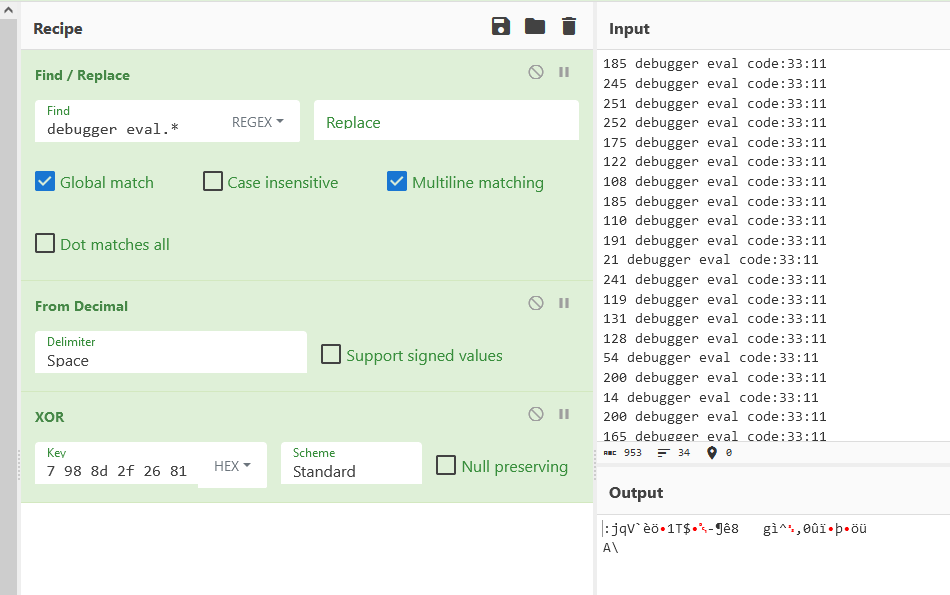Yes, I know the find and replace for debugger eval is jank but I was rushing to blood and wasn’t really thinking straight.

Anyways, the output obviously isn’t anything readable. I guess the md5 hash must have been wrong?

I opened Chrome to try to debug this time and I was surprised to see it was actually able to skip debugger statements! I put a breakpoint in the md5 hashing function only to find…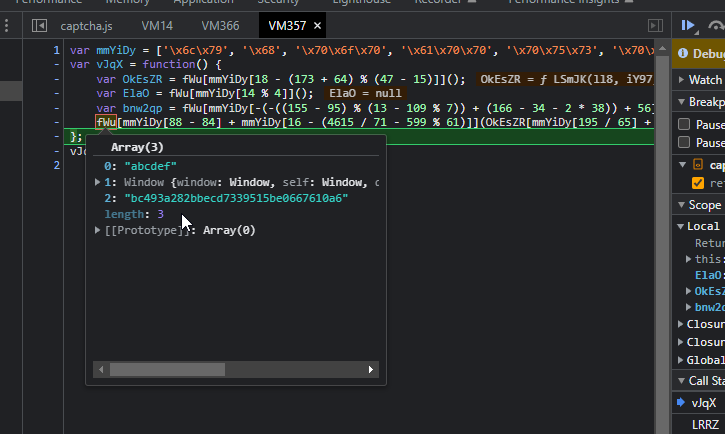It is the right hash! I almost started to redo decompiling the code until I looked at the input. It was too short compared to the data to xor.

Well as it turns out, Firefox has a bug where selecting from the console and scrolling will deselect anything that scrolls off the screen. So a small portion of the top of the console output wasn’t copied. I stored all of the xor_a values into an array and printed it all at once: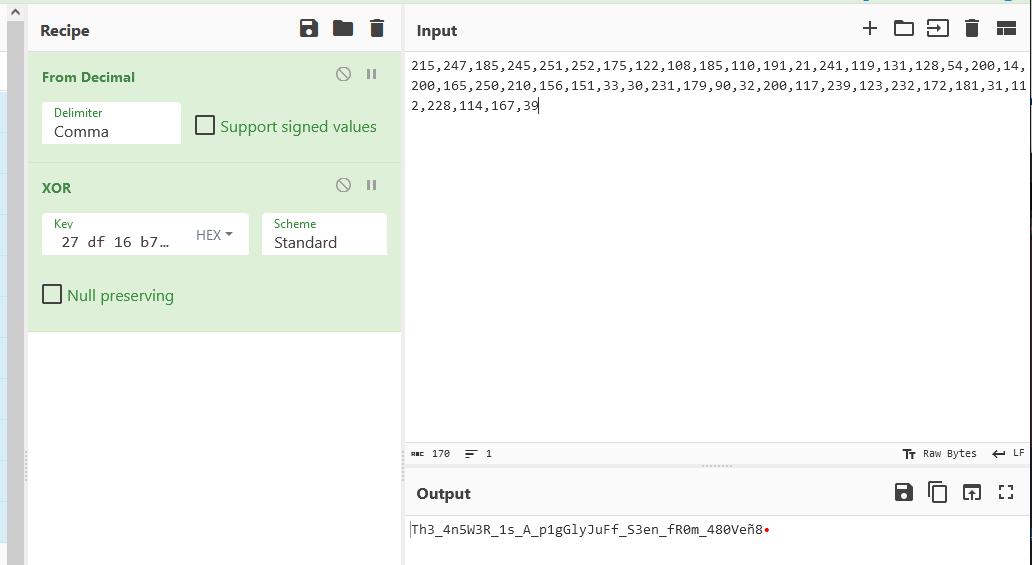Sadly I missed first blood by a mere 7 minutes. Had I only gotten up earlier that morning!

But honestly, this was probably my favorite challenge out of the bunch and it wasn’t even considered a rev 😅

Decompiler code

Disassembler code